### 4. DESCRIPTION FUNCTIONS

Principal description functions that characterize the present large-scale structure of the Universe are the power spectrum of matter and galaxies, the correlation function of galaxies and clusters, the cluster mass distribution, the void probability function (VPF), and functions based on the clustering of galaxies and clusters - the multiplicity function, and the percolation function. The structure of the early Universe can be described by the angular spectrum of the Cosmic Microwave Background (CMB). Here we discuss in more detail properties of the power spectrum and correlation function, and their dependence on geometrical properties of the distribution of galaxies and clusters.

4.1. Power spectrum

The power spectrum describes the fluctuating density field(x) through its Fourier componentsk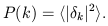(3)

Here k is the wavenumber in units of h Mpc-1. The power spectrum can be characterised by the power index on large scales, n, and by its amplitude on certain characteristic scales. For the last purpose usually very large scales (~ 1000 h-1 Mpc) are used, where the amplitude is fixed by CMB observations by the COBE satellite , and scales where the power spectrum becomes non-linear, r8 h-1 Mpc. The amplitude of the power spectrum on this scale can be expressed through the8 parameter - which denotes the rms density fluctuations within a sphere of radius 8 h-1 Mpc. It can be calculated by integrating the power spectrum of matter.

The comparison of the distribution of real galaxies and particles in simulations has shown that there are no luminous galaxies in voids; here the matter has remained in its primordial dark form. Now we consider the influence of the presence of dark matter in voids on the power spectrum of the clustered matter associated with galaxies. Low-density matter in voids forms a smooth background of almost constant density. The density contrast of matter(x) can be expressed as(x) = (D(x) - Dm) / Dm, where D(x) is the density at location x, and Dm is the mean density of matter averaged over the whole space under study. If we exclude from the sample of all particles a population of approximately constant density (void particles, see horizontal line in the right panel of Figure 2), but preserve all particles in high-density regions, then the amplitudes of absolute density fluctuations remain the same (as they are determined essentially by particles in high-density regions), but the amplitudes of relative fluctuations with respect to the mean density increase by a factor which is determined by the ratio of mean densities, i.e. by the fraction of matter in the new density field with respect to the previous one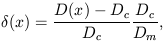(4)

here Dc / Dm = Fc is the fraction of matter in the clustered (galaxy) population. A similar formula holds for the density contrast in Fourier space, and we obtain the relation between power spectra of matter and the clustered population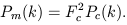(5)

We define the biasing parameter of galaxies (actually of all clustered matter associated with galaxies) relative to matter through the ratio of power spectra of galaxies and matter. Both spectra are functions of the wavenumber k, thus the biasing parameter is a function of k. Numerical simulations by Einasto  show that in the linear regime of the structure evolution the biasing parameter is practically constant for wavenumbers smaller than k0.8 h Mpc-1 (scales larger than about 8 h-1 Mpc). Its value found from simulations is very close to the expected value calculated from Equation (5). We come to the conclusion that the biasing parameter is determined by the fraction of matter in the clustered population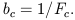(6)

A summary of recent observational data on power spectra of galaxies and clusters of galaxies of various type is shown in the left panel of Figure 3, according to a compilation by Einasto . Galaxy and cluster spectra are adjusted in amplitude to reduce them to the power spectrum of APM galaxies. We assume that the amplitude of the power spectrum of APM galaxies represents well the amplitude of the whole clustered (galaxy) population. On large scales we use a recent determination  on the basis of Abell clusters of richness class 1 and higher.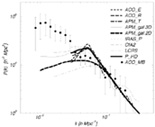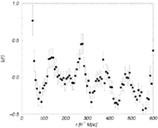Figure 3. Left: power spectra of galaxies and clusters of galaxies normalized to the amplitude of the 2-D APM galaxy power spectrum. For clarity error bars are not indicated and spectra are shown as smooth curves rather than discrete data points. Bold lines show spectra for clusters data. Points with error bars show the spectrum of Abell clusters by Miller & Batuski  adjusted to the galaxy spectrum amplitude by a relative bias factor b = 3.2. Right: correlation function of Abell clusters located in superclusters with at least 8 clusters .